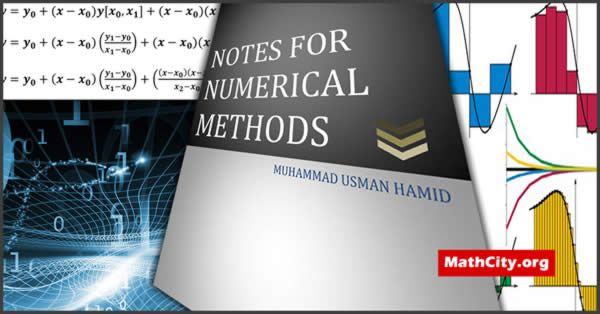# Notes for Numerical Methods by M Usman HamidThese notes are initially provided by Mr. Anwar Khan. Later the updated version is send by Muhammad Tahir. We are really very thankful to Mr. Anwar Khan and Muhammad Tahir for providing these notes and appreciates their effort to publish these notes on MathCity.org

The area of mathematics known as numerical analysis offers instruments and strategies for resolving mathematical issues in numerical form. In numerical analysis, our main areas of interest are the use and evaluation of numerical algorithms that aim to provide a rough answer to a mathematical issue.

Numerical algorithm refers to a comprehensive collection of steps that provides a rough answer to a mathematical problem.

A good numerical algorithm depends upon the following properties:

1. Number of computations i.e. Addition, Subtraction, Multiplication and Division.
2. Applicable to a class of problems.
3. Speed of convergence.
4. Error management.
5. Stability.

It covers the syllabus of Numerical Analysis paper of MSc Mathematics or a course in BS Mathematics. See the contents of the notes given below to see the topics covered by these notes.

 Name Numerical Analysis Muhammad Usman Hamid Mr. Anwar Khan 153 pages PDF (see Software section for PDF Reader) 2.30 MB
• Introduction
• Error analysis
• Solution of the non-linear equations
• Bisection methods
• False position method
• Secant method
• Fixed point iteration method
• Newton Raphson method
• Geometrical interpretation (graphics) of Newton Raphson formula
• Newton Raphson extended formula
• The solution of linear system of equations
• Gauss elimination method
• Gauss Jordan elimination method
• Matrix invertion
• Crouts reduction method
• Diagonally dominant system
• Ill condition linear system
• Jacobi’s method
• Gauss seidel iteration method
• The power method
• Difference operators
• Interpolation
• Lagrange’s interpolation formula
• Hermit interpolation
• Numarical differentiation
• Numerical integration
• Trapezoidal rule
• Simpson’s $\left(\frac{1}{3}\right)$ rule
• Simpson’s $\left(\frac{3}{8}\right)$ rule
• Weddle’s
• Boole’s rule
• Rectangular rule
• Double integration
• Newton’s Cotes formula
• Differential equations
• Methods for numerical solutions of ordinary differential equations
• Taylor’s series expansion
• Euler’s method
• Modified Euler method
• Runge Kutta methods
• Second order Runge Kutta method
• Classical Runge Kutta method (RK - method of order four)
• Predictor - corrector methods
• Milne’s method